/

### Coefficient of tail dependence of a Clayton copula

Bivariate Archimedean copulas are copulas that take the form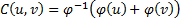for some suitable function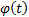where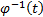is the inverse function of(not its reciprocal). A special case is the Clayton copula, which has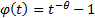where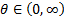.

The coefficient of (joint lower) tail dependence is defined as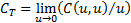. For the Clayton copula this can take any value in the range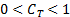. This is because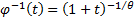since then (for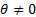):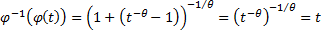Hence the (bivariate) Clayton copula is: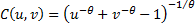.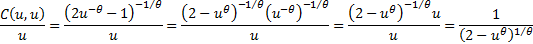So the coefficient of (joint lower) tail dependence for the (bivariate) Clayton copula is: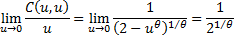As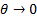this tends to 0 from above, and as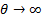this tends to 1 from below and can take any intermediate value, i.e. the range of lower tail dependences that the Clayton copula can exhibit is the range (0,1).

For an arbitrary copula the coefficient of tail dependence,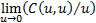, can in addition take the values 0 (e.g. the independence copula or any Gaussian copula that does not involve perfect correlation) or 1 (e.g. perfectly correlated Gaussian copula) or it may be ill-defined, i.e. it may not exist.